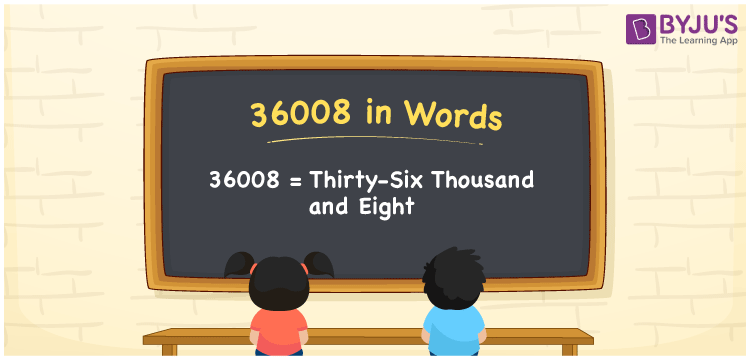# 36008 in Words

36008 in words is written as Thirty-six thousand eight. In both the International System of Numerals and the Indian System of Numerals, 36008 is written as Thirty-six thousand eight. The number 36008 is a Cardinal Number as it denotes some quantity. For example, “that mobile phone costs 36008 rupees”.

 36008 in Words Thirty-six thousand eight Thirty-six thousand eight in Number 36008

## 36008 in English Words

36008 in English words is read as “Thirty-six thousand eight”.## How to Write 36008 in Words?

To write 36008 in words, we shall use the place value chart. In the place value chart, put 3 in the ten thousands, 6 in the thousands, 0 in the hundreds and tens, and 8 in the ones. Let us make a place value chart to write the number 36008 in words.

 Ten Thousands Thousands Hundreds Tens Ones 3 6 0 0 8

Thus, we can write the expanded form as

3 × Ten Thousand + 6 × Thousand + 0 × Hundred + 0 × Ten + 8 × One

= 3 × 10000 + 6 × 1000 + 0 × 100 + 0 × 10 + 8 × 1

= 30000 + 6000 + 0 + 0 + 8

= 36008

= Thirty-six thousand eight.

36008 is a natural number, the successor of 36007 and the predecessor of 36009.

36008 in words – Thirty-six thousand eight

• Is 36008 an odd number? – No
• Is 36008 an even number? – Yes
• Is 36008 a perfect square number? – No
• Is 36008 a perfect cube number? – No
• Is 36008 a prime number? – No
• Is 36008 a composite number? – Yes

## Frequently Asked Questions on 36008 in Words

Q1

### How to write 36008 in words?

36008 in words is written as Thirty-six thousand eight.
Q2

### How to write 36008 in the International and Indian System of Numerals?

In both, the system of numerals, 36008 in words, is written as Thirty-six thousand eight.
Q3

### How to write 36008 in a place value chart?

In the place value chart, write 3 in the ten thousands, 6 in the thousands, 0 in the hundreds and tens, and 8 in the ones, respectively.